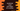# JavaScript program to mask the start digits of a phone number## JavaScript program to mask the start digits of a phone number using padStart:

In this post, we will learn how we can mask the start digits of a phone number by using padStart method in JavaScript. padStart is a useful method defined in JavaScript strings and we can use it to pad a string with another string at the beginning of the string.

Let’s have a look at this method definition and how it works.

padStart is defined as like below:

``padStart(length, string)``

Here,

• length is the length of the final string after the padding is done. If the length is less than the size of the current string, then it will return the same string without any change.
• string is the string to use for padding. If its size is too long, it is truncated from the end.

It returns the final padded string.

Using padStart, we can mask the start digits of a phone number. For example, if the phone number is of 10 digits, we can get the last 4 digits of the number and using padStart, we can fill the first digits to any other character like *.

So,

• Get the last 4 digits of the phone number
• Using padStart create a new string equal to the size of the phone number and add * to the start of the string.

## JavaScript program:

Below is the complete program:

``````const getMaskedNumber = (number) => {
const endDigits = number.slice(-4);